# Java Program to Reverse a String using Recursion

In this tutorial you will learn about the Java Program to Reverse a String using Recursion and its application with practical example.

## Java Program to Reverse a String using Recursion

In this tutorial, we will learn to create a Java program that will reverse a string Using Recursion in Java programming.

## Prerequisites

Before starting with this tutorial, we assume that you are the best aware of the following Java programming topics:

• Operators in Java Programming.
• Usage of Conditional statements in Java Programming.
• Basic Input and Output function in Java Programming.
• Basic Java programming.

## What is Recursion? Reverse a Sentence Using it?

The recursion means a function or program that repeats itself until the condition is satisfied. The recursion repeats the program execution in a controlled format. Today we will reverse a sentence using the recursion.

## Algorithm:-

In this program of recursion, we will take the input string from the user and store it in the string variable. Then will use that string variable in a user-defined function. At last, we will reverse that string using a recursion program.

With the help of the below, code, we will reverse a string Using Recursion.

## Output:-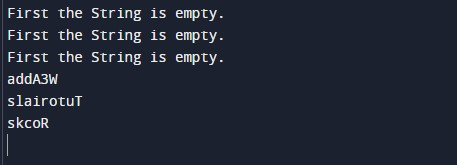In the above program, we have first initialized the required variable.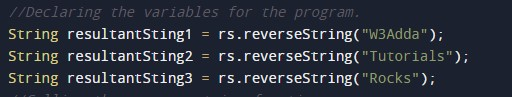• ResultantString1 = it will hold the string value for the program.
• ResultantString2 = it will hold the string value for the program.
• ResultantString3 = it will hold the string value for the program.

Calling the reverse string function.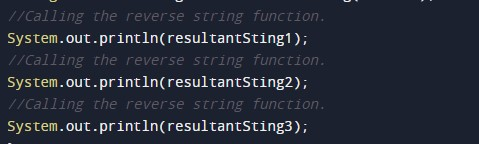Body of the Reverse string function.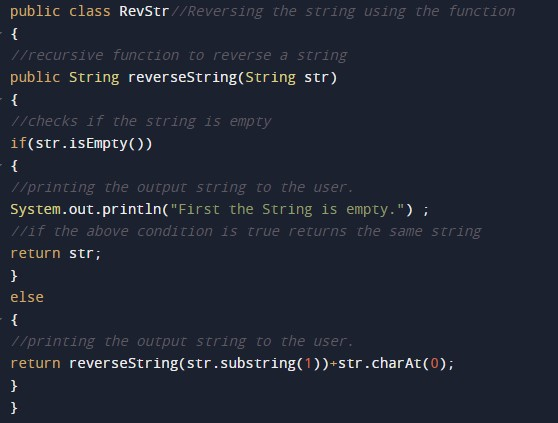Printing the output of the program reversed string.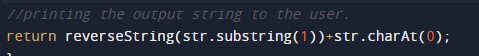In this tutorial we have learn about the Java Program to Reverse a String using Recursion and its application with practical example. I hope you will like this tutorial.